SLVSCS1B March   2015  – May 2015

PRODUCTION DATA.

1. Features
2. Applications
3. Description
4. Simplified Schematic
5. Revision History
6. Pin Configuration and Functions
7. Specifications
8. Detailed Description
1. 8.1 Overview
2. 8.2 Functional Block Diagram
3. 8.3 Feature Description
4. 8.4 Device Functional Modes
9. Application and Implementation
1. 9.1 Application Information
2. 9.2 Typical Application
1. 9.2.1 Half-Bridge LLC
1. 9.2.1.1 Design Requirements
2. 9.2.1.2 Detailed Design Procedure
3. 9.2.1.3 Application Curves
10. 10Power Supply Recommendations
11. 11Layout
12. 12Device and Documentation Support
13. 13Mechanical, Packaging, and Orderable Information

• DRS|6
• DRS|6

## 7 Specifications

### 7.1 Absolute Maximum Ratings

over operating free-air temperature range (unless otherwise noted) (1)
MIN MAX UNIT
Input voltage VCC –0.3 20 V
IN, CRTL –0.3 3.8
VD –1 45
Maximum VCC continuous input current DC current 50 mA
Output current, peak (pulse) 6 A
Switching frequency, ƒS 2000 kHz
Operating junction temperature, TJ –40 125 °C
Lead temperature, soldering, 10 s, T(SOL) 300 °C
Storage temperature, Tstg –65 150 °C
(1) Stresses beyond those listed under Absolute Maximum Ratings may cause permanent damage to the device. These are stress ratings only, which do not imply functional operation of the device at these or any other conditions beyond those indicated under Recommended Operating Conditions. Exposure to absolute-maximum-rated conditions for extended periods may affect device reliability.

### 7.2 ESD Ratings

VALUE UNIT
V(ESD) Electrostatic discharge Human body model (HBM), per ANSI/ESDA/JEDEC JS–001, all pins(1) ±2000 V
Charged device model (CDM), per JEDEC specification JESD22-C101, all pins(2) ±500
(1) JEDEC document JEP155 states that 500-V HBM allows safe manufacturing with a standard ESD control process.
(2) JEDEC document JEP157 states that 250-V CDM allows safe manufacturing with a standard ESD control process.

### 7.3 Recommended Operating Conditions

over operating free-air temperature range (unless otherwise noted)
MIN NOM MAX UNIT
VCC VCC input voltage from a low impedance source 4.5 18 V
VIN Input voltage 0 3.6 V
C(BP) VCC bypass capacitor 1 µF
TJ Operating junction temperature –40 125 °C

### 7.4 Thermal Information

THERMAL METRIC(1) UCD7138 UNIT
DRS (WSON)
6 PINS
RθJA Junction-to-ambient thermal resistance 73.4 °C/W
RθJC(top) Junction-to-case (top) thermal resistance 84.2
RθJB Junction-to-board thermal resistance 46.3
ψJT Junction-to-top characterization parameter 2.6
ψJB Junction-to-board characterization parameter 46.4
RθJC(bot) Junction-to-case (bottom) thermal resistance 12.4
(1) For more information about traditional and new thermal metrics, see the Semiconductor and IC Package Thermal Metrics application report, SPRA953.

### 7.5 Electrical Characteristics

At VCC = 12 VDC, –40°C < TJ = TA < 125°C, 1µF capacitor from VCC to GND, all voltages are with respect to ground and currents are positive into and negative out of the specified terminal, unless otherwise noted. Typical values are at TJ = 25°C.
PARAMETER TEST CONDITIONS MIN TYP MAX UNIT
VCC BIAS SUPPLY
ICC(UV) VCC current, undervoltage VCC = 3.4 V 122 186 µA
ICC(ON) VCC current, no switching VCC = 12 V 0.85 1.1 mA
ICC(OPERATE) VCC current, normal operation (1) VCC = 12 V, C(LOAD) = 10 nF,
ƒ = 100 kHz
13 17 mA
GATE INPUT (IN)
VIH Input signal high threshold 1.93 2.03 2.10 V
VIL Input signal low threshold 0.98 1.03 1.08 V
VI(hys) Input hysteresis 0.90 1.00 V
DTC OUTPUT
VOL(DTC) Low level output voltage 0.25 V
VOH(DTC) High level output voltage 2.5 V
IOH(DTC) Output sinking current 4 mA
IOL(DTC) Output sourcing current –4 mA
VDTC Maximum DTC pin output voltage 3.5 3.6 V
UNDERVOLTAGE LOCKOUT SECTION (UVLO)
VCC(ON) VCC turnon threshold 3.30 3.80 4.30 V
VCC(OFF) VCC turnoff threshold 3.10 3.56 4.02 V
VCC(hys) UVLO hysteresis VCC(hys) = VCC(ON) – VCC(OFF) 0.24 V
COMPARATOR
VTH Body diode conduction sensing threshold –179 –147 –113 mV
CI(VD–ground) Differential input capacitance between VD and ground (1) VD = –150 mV 20 pF
GATE DRIVER
VCC-VOH Output high voltage IOUT = –10 mA 0.038 0.064 V
VOL Output low voltage IOUT = 10 mA 0.0025 0.009 V
R(UP) Pullup resistance TA = 25°C, IOUT = –25 mA to
–50 mA
5 6.1 Ω
TA = –40°C to 125°C,
IOUT = –50 mA
5 6.3 Ω
R(DOWN) Pulldown resistance TA = 25°C, IOUT = 25 mA to
50 mA
0.31 0.44 Ω
TA = –40°C to 125°C,
IOUT = 50 mA
0.33 0.45 Ω
IO(source) Output peak current (source) (1) C(LOAD) = 0.22 µF, ƒS = 1 kHz,
5-V output
–4 A
IO(sink) Output peak current (sink) (1) C(LOAD) = 0.22 µF, ƒS = 1 kHz,
5-V output
6 A
(1) Ensured by Design, not tested in Production.

### 7.6 Switching Characteristics

At VCC = 12 VDC, –40°C < TJ = TA < 125°C, 1µF capacitor from VCC to GND, all voltages are with respect to ground and currents are positive into and negative out of the specified terminal, unless otherwise noted. Typical values are at TJ = 25°C.
PARAMETER TEST CONDITIONS MIN TYP MAX UNIT
tr Rise time C(LOAD) =1 nF, VCC = 5 V,
See Figure 1 and Figure 24
5 8 ns
C(LOAD) =1 nF, VCC = 12 V,
See Figure 1 and Figure 24
4 8 ns
tf Fall time C(LOAD)=1 nF, VCC = 5 V,
See Figure 1 and Figure 24
3.36 5 ns
C(LOAD)=1 nF, VCC = 12 V,
See Figure 1 and Figure 24
3.5 5 ns
tw(VD) Minimum VD pulse duration (width) that changes the DTC output state VCC = 5 V 10 23 ns
VCC = 12 V 10 23 ns
tw(IN) Minimum IN duration (width) that changes OUT state VCC = 5 V 11 13 ns
VCC = 12 V 11 13 ns
td(1) Gate driver turn on propagation delay C(LOAD) = 1 nF, VIN = 0 V to 3.3 V, VCC = 5 V,
VVD = –0.5 V, See Figure 1 and Figure 24
14 26.6 ns
C(LOAD) = 1 nF, VIN = 0 V to 3.3 V, VCC = 12 V,
VVD = –0.5 V, See Figure 1 and Figure 24
14 25 ns
td(2) Gate driver turn off propagation delay C(LOAD) = 1 nF, VIN = 3.3 V to 0 V, VCC = 5 V,
See Figure 1 and Figure 24
14 22.9 ns
C(LOAD) = 1 nF, VIN = 3.3 V to 0 V, VCC = 12 V,
See Figure 1 and Figure 24
14 22 ns
td(COMP) Body-diode conduction detection-comparator controlled-turnon propagation delay(1) C(LOAD) = 1 nF, VIN = 3.3 V, VCC = 5 V, VVD = 2 V to –0.5 V, See Figure 24 28 36 ns
C(LOAD) = 1 nF, VIN = 3.3 V, VCC = 12 V, VVD = 2 V to –0.5 V, See Figure 24 26 33 ns
td(DTC) DTC output propagation delay VCC = 5 V 21 27 ns
VCC = 12 V 18 25 ns
(1) For details about the operation, see the Gate Turnon and Turnoff section.Figure 1. Input Driver Operation

### 7.7 Typical CharacteristicsVCC = 3.4 V
Figure 2. Start-Up Current (ICC(UV)) vs Temperature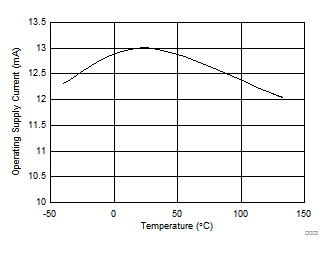VCC = 12 V C(LOAD) = 10 nF ƒ = 100 kHz
Figure 4. Operating Supply current (ICC(OPERATE)) vs TemperatureFigure 6. UVLO On-Voltage (VCC(ON))vs Temperature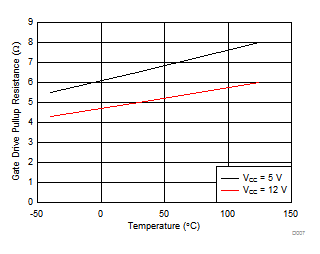Figure 8. Gate-Driver Pullup Resistance (R(UP)) vs Temperature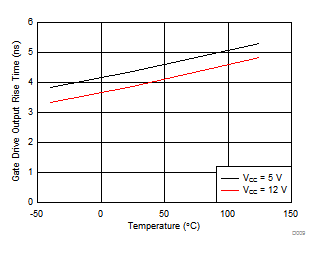Figure 10. Gate-Drive Output Rise Time (tr) vs Temperature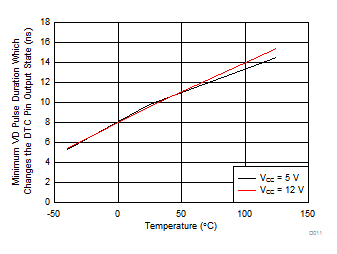Figure 12. Minimum VD Pulse Duration Which Changes the DTC Pin Output State (tw(VD)) vs Temperature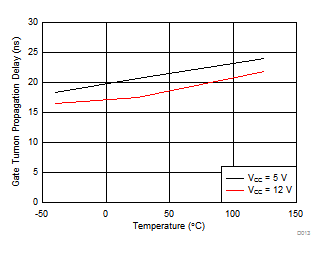Figure 14. Gate Turnon Propagation Delay (td(1)) vs TemperatureFigure 16. Comparator-Controlled Turnon Delay for Body-Diode Conduction Detection (td(COMP)) vs Temperature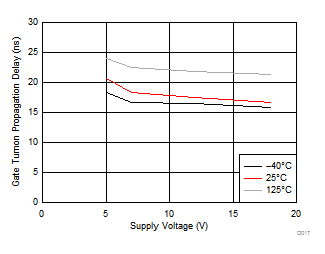Figure 18. Gate Turnon Propagation Delay (td(1)) vs Supply Voltage (VCC)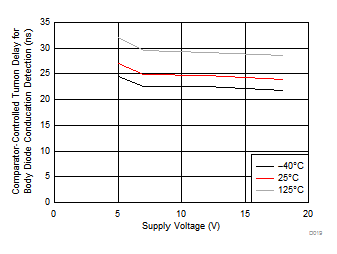Figure 20. Comparator-Controlled Turnon Delay for Body-Diode Conduction Detection (td(COMP)) vs Supply Voltage (VCC)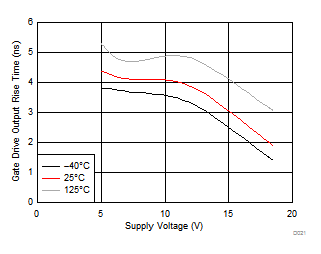Figure 22. Gate-Drive Output Rise Time (tr) vs Supply Voltage (VCC)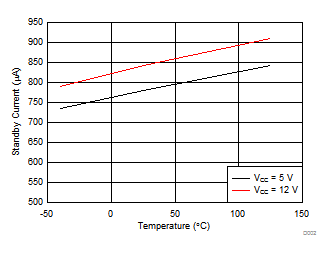No switching
Figure 3. Standby Current (ICC(ON))vs TemperatureVCC = 12 V
Figure 5. Body-Diode Conduction Sensing Threshold (VTH) vs TemperatureFigure 7. UVLO OFF-Voltage (VCC(OFF)) vs Temperature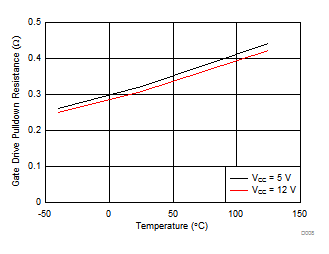Figure 9. Gate-Driver Pulldown Resistance (R(DOWN)) vs TemperatureFigure 11. Gate-Drive Output Rise Time (tf) vs TemperatureFigure 13. Minimum IN Pulse Duration Which Changes the Pin Output State (tw(IN)) vs Temperature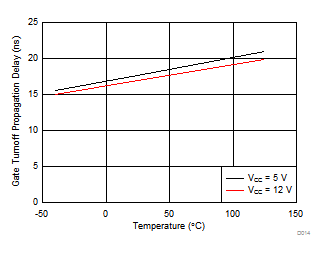Figure 15. Gate Turnoff Propagation Delay (td(2)) vs TemperatureFigure 17. DTC Output Propagation Delay (td(DTC)) vs TemperatureFigure 19. Gate Turnoff Propagation Delay (td(2)) vs Supply Voltage (VCC)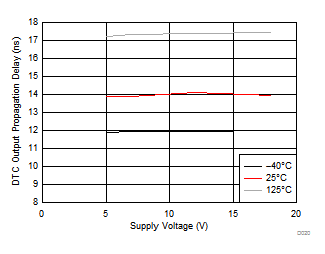Figure 21. DTC Output Propagation Delay (td(DTC)) vs Supply Voltage (VCC)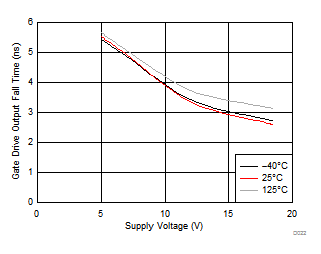Figure 23. Gate-Drive Output Fall time (tf) vs Supply Voltage (VCC)